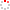#CodeStepByStep## byAge

Language/Type: Kotlin collections map

Write a function named `byAge` that accepts three parameters: 1) a `Map` where each key is a person's name (a string) and the associated value is that person's age (an integer); 2) an integer for a minimum age; and 3) an integer for a max age. Your function should return a new sorted `Map` with information about people with ages between the min and max, inclusive.

In your result map, each key is an integer age, and the value for that key is a string with the names of all people at that age, separated by "and" if there is more than one person of that age. The order of names for a given age should be listed in alphabetical order, such as "Bob and Carl" rather than "Carl and Bob". (You'll need to forcibly iterate over the parameter map in ABC order to achieve this.) Include only ages between the min and max inclusive, where there is at least one person of that age in the parameter map. If the map passed is empty, or if there are no people in the map between the min/max ages, return an empty map.

For example, if a map named `ages` stores the following key/value pairs:

```{"Allison"=18, "Benson"=48, "David"=20, "Erik"=20, "Galen"=15, "Grace=25,
"Helene"=40, "Janette"=18, "Jessica"=35, "Marty"=35, "Paul"=28, "Sara"=15,
"Stuart"=98, "Tyler"=6, "Zack"=20}
```

The call of `byAge(ages, 16, 25)` should return the following map:

```{18="Allison and Janette", 20="David and Erik and Zack", 25="Grace"}
```

For the same map, the call of `byAge(ages, 20, 40)` should return the following map:

```{20="David and Erik and Zack", 25="Grace", 28="Paul", 35="Jessica and Marty", 40="Helene"}
```
Type your Kotlin solution code here:

This is a function exercise. Write a Kotlin function as described. Do not write a complete program; just the function(s) above.